Open in App
Not now

# Ukkonen’s Suffix Tree Construction – Part 3

• Difficulty Level : Hard
• Last Updated : 04 Nov, 2022

Ukkonen’s Suffix Tree Construction – Part 1
Ukkonen’s Suffix Tree Construction – Part 2

Please go through Part 1 and Part 2, before looking at current article, where we have seen few basics on suffix tree, high level ukkonen’s algorithm, suffix link and three implementation tricks.

Here we will take string S = “abcabxabcd” as an example and go through all the things step by step and create the tree.
We will add \$ (discussed in Part 1 why we do this) so string S would be “abcabxabcd\$”.

While building suffix tree for string S of length m:

• There will be m phases 1 to m (one phase for each character)
In our current example, m is 11, so there will be 11 phases.
• First phase will add first character ‘a’ in the tree, second phase will add second character ‘b’ in tree, third phase will add third character ‘c’ in tree, ……, mth phase will add mth character in tree (This makes Ukkonen’s algorithm an online algorithm)
• Each phase i will go through at-most i extensions (from 1 to i). If current character being added in tree is not seen so far, all i extensions will be completed (Extension Rule 3 will not apply in this phase). If current character being added in tree is seen before, then phase i will complete early (as soon as Extension Rule 3 applies) without going through all i extensions
• There are three extension rules (1, 2 and 3) and each extension j (from 1 to i) of any phase i will adhere to one of these three rules.
• Rule 1 adds a new character on existing leaf edge
• Rule 2 creates a new leaf edge (And may also create new internal node, if the path label ends in between an edge)
• Rule 3 ends the current phase (when current character is found in current edge being traversed)
• Phase 1 will read first character from the string, will go through 1 extension.
(In figures, we are showing characters on edge labels just for explanation, while writing code, we will only use start and end indices – The Edge-label compression discussed in Part 2)
Extension 1 will add suffix “a” in tree. We start from root and traverse path with label ‘a’. There is no path from root, going out with label ‘a’, so create a leaf edge (Rule 2).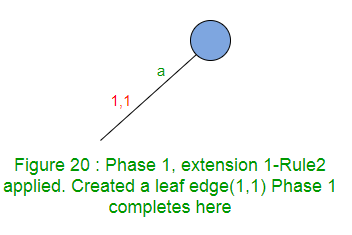Phase 1 completes with the completion of extension 1 (As a phase i has at most i extensions)
For any string, Phase 1 will have only one extension and it will always follow Rule 2.
• Phase 2 will read second character, will go through at least 1 and at most 2 extensions.
In our example, phase 2 will read second character ‘b’. Suffixes to be added are “ab” and “b”.
Extension 1 adds suffix “ab” in tree.
Path for label ‘a’ ends at leaf edge, so add ‘b’ at the end of this edge.
Extension 1 just increments the end index by 1 (from 1 to 2) on first edge (Rule 1).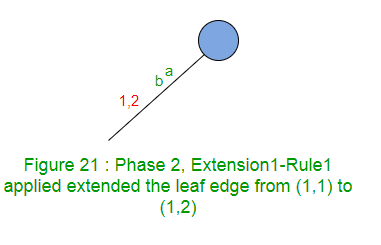Extension 2 adds suffix “b” in tree. There is no path from root, going out with label ‘b’, so creates a leaf edge (Rule 2).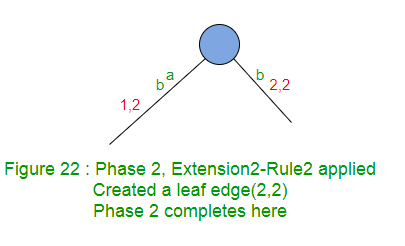Phase 2 completes with the completion of extension 2.
Phase 2 went through two extensions here. Rule 1 applied in 1st Extension and Rule 2 applied in 2nd Extension.
• Phase 3 will read third character, will go through at least 1 and at most 3 extensions.
In our example, phase 3 will read third character ‘c’. Suffixes to be added are “abc”, “bc” and “c”.
Extension 1 adds suffix “abc” in tree.
Path for label ‘ab’ ends at leaf edge, so add ‘c’ at the end of this edge.
Extension 1 just increments the end index by 1 (from 2 to 3) on this edge (Rule 1).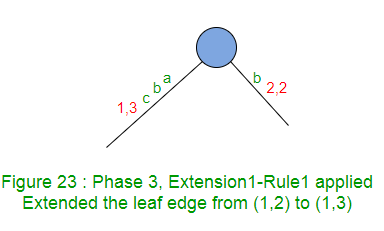Extension 2 adds suffix “bc” in tree.
Path for label ‘b’ ends at leaf edge, so add ‘c’ at the end of this edge.
Extension 2 just increments the end index by 1 (from 2 to 3) on this edge (Rule 1).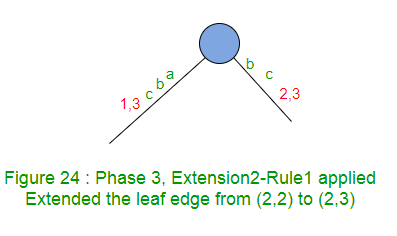Extension 3 adds suffix “c” in tree. There is no path from root, going out with label ‘c’, so creates a leaf edge (Rule 2).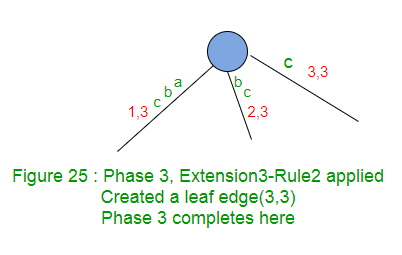Phase 3 completes with the completion of extension 3.
Phase 3 went through three extensions here. Rule 1 applied in first two Extensions and Rule 2 applied in 3rd Extension.
• Phase 4 will read fourth character, will go to at least 1 and at most 4 extensions.
In our example, phase 4 will read fourth character ‘a’. Suffixes to be added are “abca”, “bca”, “ca” and “a”.
Extension 1 adds suffix “abca” in tree.
Path for label ‘abc’ ends at leaf edge, so add ‘a’ at the end of this edge.
Extension 1 just increments the end index by 1 (from 3 to 4) on this edge (Rule 1).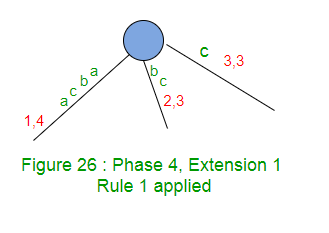Extension 2 adds suffix “bca” in tree.
Path for label ‘bc’ ends at leaf edge, so add ‘a’ at the end of this edge.
Extension 2 just increments the end index by 1 (from 3 to 4) on this edge (Rule 1).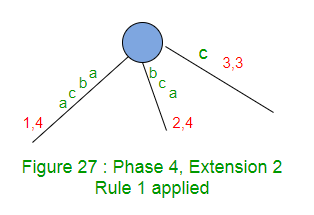Extension 3 adds suffix “ca” in tree.
Path for label ‘c’ ends at leaf edge, so add ‘a’ at the end of this edge.
Extension 3 just increments the end index by 1 (from 3 to 4) on this edge (Rule 1).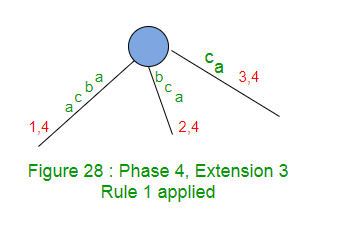Extension 4 adds suffix “a” in tree.
Path for label ‘a’ exists in the tree. No more work needed and Phase 4 ends here (Rule 3 and Trick 2). This is an example of implicit suffix tree. Here suffix “a” is not seen explicitly (because it doesn’t end at a leaf edge) but it is in the tree implicitly. So there is no change in tree structure after extension 4. It will remain as above in Figure 28.
Phase 4 completes as soon as Rule 3 is applied while Extension 4.
Phase 4 went through four extensions here. Rule 1 applied in first three Extensions and Rule 3 applied in 4th Extension.

Now we will see few observations and how to implement those.

1. At the end of any phase i, there are at most i leaf edges (if ith character is not seen so far, there will be i leaf edges, else there will be less than i leaf edges).
e.g. After phases 1, 2 and 3 in our example, there are 1, 2 and 3 leaf edges respectively, but after phase 4, there are 3 leaf edges only (not 4).
2. After completing phase i, “end” indices of all leaf edges are i. How do we implement this in code? Do we need to iterate through all those extensions, find leaf edges by traversing from root to leaf and increment the “end” index? Answer is “NO”.
For this, we will maintain a global variable (say “END”) and we will just increment this global variable “END” and all leaf edge end indices will point to this global variable. So this way, if we have j leaf edges after phase i, then in phase i+1, first j extensions (1 to j) will be done by just incrementing variable “END” by 1 (END will be i+1 at the point).
Here we just implemented the trick 3 – Once a leaf, always a leaf. This trick processes all the j leaf edges (i.e. extension 1 to j) using rule 1 in a constant time in any phase. Rule 1 will not apply to subsequent extensions in the same phase. This can be verified in the four phases we discussed above. If at all Rule 1 applies in any phase, it only applies in initial few phases continuously (say 1 to j). Rule 1 never applies later in a given phase once Rule 2 or Rule 3 is applied in that phase.
3. In the example explained so far, in each extension (where trick 3 is not applied) of any phase to add a suffix in tree, we are traversing from root by matching path labels against the suffix being added. If there are j leaf edges after phase i, then in phase i+1, first j extensions will follow Rule 1 and will be done in constant time using trick 3. There are i+1-j extensions yet to be performed. For these extensions, which node (root or some other internal node) to start from and which path to go? Answer to this depends on how previous phase i is completed.
If previous phase i went through all the i extensions (when ith character is unique so far), then in next phase i+1, trick 3 will take care of first i suffixes (the i leaf edges) and then extension i+1 will start from root node and it will insert just one character [(i+1)th] suffix in tree by creating a leaf edge using Rule 2.
If previous phase i completes early (and this will happen if and only if rule 3 applies – when ith character is already seen before), say at jth extension (i.e. rule 3 is applied at jth extension), then there are j-1 leaf edges so far.
We will state few more facts (which may be a repeat, but we want to make sure it’s clear to you at this point) here based on discussion so far:

• Phase 1 starts with Rule 2, all other phases start with Rule 1
• Any phase ends with either Rule 2 or Rule 3
• Any phase i may go through a series of j extensions (1 <= j <= i). In these j extensions, first p (0 <= p < i) extensions will follow Rule 1, next q (0 <= q <= i-p) extensions will follow Rule 2 and next r (0<= r <= 1) extensions will follow Rule 3. The order in which Rule 1, Rule 2 and Rule 3 apply, is never intermixed in a phase. They apply in order of their number (if at all applied), i.e. in a phase, Rule 1 applies 1st, then Rule 2 and then Rule 3
• In a phase i, p + q + r <= i
• At the end of any phase i, there will be p+q leaf edges and next phase i+1 will go through Rule 1 for first p+q extensions

In the next phase i+1, trick 3 (Rule 1) will take care of first j-1 suffixes (the j-1 leaf edges), then extension j will start where we will add jth suffix in tree. For this, we need to find the best possible matching edge and then add new character at the end of that edge. How to find the end of best matching edge? Do we need to traverse from root node and match tree edges against the jth suffix being added character by character? This will take time and overall algorithm will not be linear. activePoint comes to the rescue here.
In previous phase i, while jth extension, path traversal ended at a point (which could be an internal node or some point in the middle of an edge) where ith character being added was found in tree already and Rule 3 applied, jth extension of phase i+1 will start exactly from the same point and we start matching path against (i+1)th character. activePoint helps to avoid unnecessary path traversal from root in any extension based on the knowledge gained in traversals done in previous extension. There is no traversal needed in 1st p extensions where Rule 1 is applied. Traversal is done where Rule 2 or Rule 3 gets applied and that’s where activePoint tells the starting point for traversal where we match the path against the current character being added in tree. Implementation is done in such a way that, in any extension where we need a traversal, activePoint is set to right location already (with one exception case APCFALZ discussed below) and at the end of current extension, we reset activePoint as appropriate so that next extension (of same phase or next phase) where a traversal is required, activePoint points to the right place already.

activePoint: This could be root node, any internal node or any point in the middle of an edge. This is the point where traversal starts in any extension. For the 1st extension of phase 1, activePoint is set to root. Other extension will get activePoint set correctly by previous extension (with one exception case APCFALZ discussed below) and it is the responsibility of current extension to reset activePoint appropriately at the end, to be used in next extension where Rule 2 or Rule 3 is applied (of same or next phase).
To accomplish this, we need a way to store activePoint. We will store this using three variables: activeNode, activeEdge, activeLength.
activeNode: This could be root node or an internal node.
activeEdge: When we are on root node or internal node and we need to walk down, we need to know which edge to choose. activeEdge will store that information. In case, activeNode itself is the point from where traversal starts, then activeEdge will be set to next character being processed in next phase.
activeLength: This tells how many characters we need to walk down (on the path represented by activeEdge) from activeNode to reach the activePoint where traversal starts. In case, activeNode itself is the point from where traversal starts, then activeLength will be ZERO.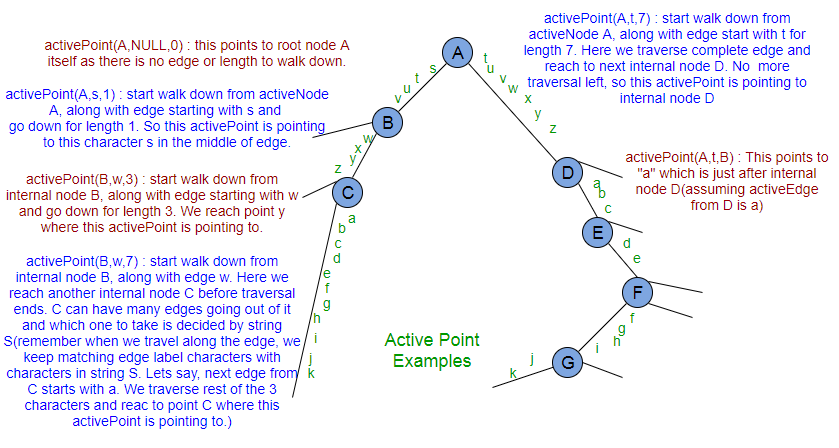After phase i, if there are j leaf edges then in phase i+1, first j extensions will be done by trick 3. activePoint will be needed for the extensions from j+1 to i+1 and activePoint may or may not change between two extensions depending on the point where previous extension ends.

activePoint change for extension rule 3 (APCFER3): When rule 3 applies in any phase i, then before we move on to next phase i+1, we increment activeLength by 1. There is no change in activeNode and activeEdge. Why? Because in case of rule 3, the current character from string S is matched on the same path represented by current activePoint, so for next activePoint, activeNode and activeEdge remain the same, only activeLenth is increased by 1 (because of matched character in current phase). This new activePoint (same node, same edge and incremented length) will be used in phase i+1.

activePoint change for walk down (APCFWD): activePoint may change at the end of an extension based on extension rule applied. activePoint may also change during the extension when we do walk down. Let’s consider an activePoint is (A, s, 11) in the above activePoint example figure. If this is the activePoint at the start of some extension, then while walk down from activeNode A, other internal nodes will be seen. Anytime if we encounter an internal node while walk down, that node will become activeNode (it will change activeEdge and activeLenght as appropriate so that new activePoint represents the same point as earlier). In this walk down, below is the sequence of changes in activePoint:
(A, s, 11) — >>> (B, w, 7) —- >>> (C, a, 3)
All above three activePoints refer to same point ‘c’
Let’s take another example.
If activePoint is (D, a, 11) at the start of an extension, then while walk down, below is the sequence of changes in activePoint:
(D, a, 10) — >>> (E, d, 7) — >>> (F, f, 5) — >> (G, j, 1)
All above activePoints refer to same point ‘k’.
If activePoints are (A, s, 3), (A, t, 5), (B, w, 1), (D, a, 2) etc when no internal node comes in the way while walk down, then there will be no change in activePoint for APCFWD.
The idea is that, at any time, the closest internal node from the point, where we want to reach, should be the activePoint. Why? This will minimize the length of traversal in the next extension.

activePoint change for Active Length ZERO (APCFALZ): Let’s consider an activePoint (A, s, 0) in the above activePoint example figure. And let’s say current character being processed from string S is ‘x’ (or any other character). At the start of extension, when activeLength is ZERO, activeEdge is set to the current character being processed, i.e. ‘x’, because there is no walk down needed here (as activeLength is ZERO) and so next character we look for is current character being processed.

4. While code implementation, we will loop through all the characters of string S one by one. Each loop for ith character will do processing for phase i. Loop will run one or more time depending on how many extensions are left to be performed (Please note that in a phase i+1, we don’t really have to perform all i+1 extensions explicitly, as trick 3 will take care of j extensions for all j leaf edges coming from previous phase i). We will use a variable remainingSuffixCount, to track how many extensions are yet to be performed explicitly in any phase (after trick 3 is performed). Also, at the end of any phase, if remainingSuffixCount is ZERO, this tells that all suffixes supposed to be added in tree, are added explicitly and present in tree. If remainingSuffixCount is non-zero at the end of any phase, that tells that suffixes of that many count are not added in tree explicitly (because of rule 3, we stopped early), but they are in tree implicitly though (Such trees are called implicit suffix tree). These implicit suffixes will be added explicitly in subsequent phases when a unique character comes in the way.

We will continue our discussion in Part 4 and Part 5. Code implementation will be discussed in Part 6.